# Acetonitrile (CH3CN) lewis dot structure, molecular geometry, polar or non-polar, hybridization

Home  > Chemistry Article > CH3CN lewis structure and its molecular geometry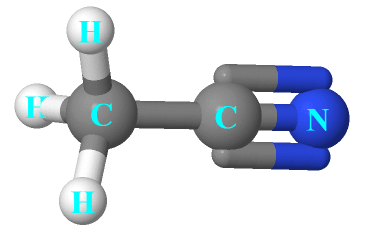Acetonitrile also called cyanomethane or methyl cyanide is a chemical compound with the molecular formula CH3CN. It is a colorless liquid and has a fruity odor. It is mainly used as a polar aprotic solvent or as a solvent in the purification of butadiene in refineries.

In this tutorial, we will discuss CH3CN lewis structure, molecular geometry, polar or nonpolar, hybridization, etc.

Acetonitrile is toxic by inhalation and causes irritation of mucous membranes. It can also cause chronic health conditions in the central nervous system, severe headaches, and numbness, etc.

Properties of Acetonitrile

• It has a molar mass of 41.053 g·mol−1.
• It appears as a colorless liquid and is less dense than water.
• It has a boiling point of 81.3 to 82.1 °C and a melting point of −46 to −44 °C.
• It is miscible in water.
• It is an aliphatic nitrile and a volatile organic compound.
 Name of Molecule Acetonitrile/methyl cyanide Chemical formula CH3CN Molecular geometry of CH3CN Tetrahedral/linear Electron geometry of CH3CN Tetrahedral/linear Hybridization Sp³ and Sp Nature polar molecule Total Valence electron in CH3CN lewis structure 16
Page Contents

## How to draw CH3CN lewis structure?

CH3CN lewis’s structure is very interesting to draw as this structure contains one triple bond, four single bonds, and two central atoms. There is one lone pair on the nitrogen atom and a total of 7 bonded pairs present in the CH3CN lewis structure.

Let’s start the construction of its lewis diagram step by step-

## Follow some steps for drawing the lewis structure of CH3CN

1. Count total valence electron in CH3CN

First, determine the total valence electron in the CH3CN molecule, to do this, look at the periodic group of each atom of CH3CN.

The carbon atom belongs to the 14th periodic group, nitrogen to the 15th group, and hydrogen to the 1st periodic group. Hence, the valence electron in a carbon atom is 4, in nitrogen, it is 5, and hydrogen has only 1 valence electron in its outermost shell.

⇒ Total number of the valence electrons in carbon = 4

⇒ Total number of the valence electrons in nitrogen = 5

⇒ Total number of the valence electrons in hydrogen = 1

∴ Total number of valence electron available for CH3CN lewis structure = 4 + 1(3) + 4 + 5 = 16 valence electrons         [∴CH3CN molecule has two carbon, three hydrogen atom, and one nitrogen]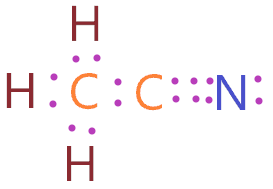2. Find the least electronegative atom and placed it at center

The lesser the electronegativity of an atom more is the ability to share electrons, hence, the least electronegative always takes the central place in the lewis diagram.

In the case of the CH3CN molecule, hydrogen always goes outside as it can share only a maximum of two electrons, from carbon and nitrogen atom, carbon is the less electronegative atom.

Therefore, put the two carbon atoms adjacent to each other in a central position, and spread the three hydrogens around one carbon atom(CH3), and set the nitrogen atom adjacent to other carbon(C≡N).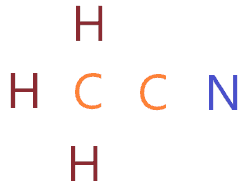3. Connect outer atoms to central atom with a single bond

Now connect each outer atom to the central atom with a single bond. Simply put the single bond in between each atom in 2nd step structure.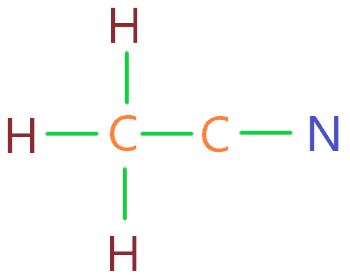As you see in the above figure, there is a total of 5 single bonds are place in between the atoms. One single bond contains two electrons, so, these 5 bonds contain a total of 10 valence electrons.

This means we used 10 valence electrons in the above structure from a total of 16 available valence electrons for drawing the CH3CN lewis structure.

∴ (16 – 10) = 6 valence electrons

So, we are left with 6 valence electrons more.

4. Place remaining valence electrons starting from outer atom first

The outer atom in the CH3CN molecule is hydrogen and nitrogen, since, hydrogen atom only needs two electrons for completing the octet and it has already two electrons because of the single bond attached to it.

So, place the remaining 6 valence electrons around the nitrogen atom to complete its octet.By looking at the above figure, we see nitrogen atom completed its octet as it has 8 valence electrons in the outermost shell, hydrogen atoms also completed their octet as they only need two electrons which are fulfilled by a single bond attached to it.

The left side carbon atom also completed its octet as it is sharing 8 electrons with the help of 4 single bonds, but the right side carbon atom has only 4 electrons(2 single bonds).

So, it needs 4 more valence electrons to complete the octet.

Let’s complete its desire in the next step-

5. Complete central atom octet and make covalent bond if necessary

The only right side carbon atom is left without the octet. To completing the octet of right side carbon, convert the two lone pairs of nitrogen atoms into a covalent bond.As you see in the above figure, we have successfully converted the lone pair of nitrogen atoms into covalent bonds without violating the octet. Now right side carbon atom is also completed its octet as it has 8 electrons to share(one triple bond contains 6 electrons).

So, all the atoms fulfilled the octet comfortably, now just check for the formal charge to know if the above CH3CN lewis structure is stable or not.

6. Check the stability with the help of a formal charge concept

To determine the formal charge on an atom. Use the formula given below-

⇒ Formal charge = (valence electrons – lone pair electrons –  1/2 bonded pair electrons)

For hydrogen atom:

⇒ Valence electrons of hydrogen = 1

⇒ Lone pair electrons on hydrogen = 0

⇒ Shared pair electrons around hydrogen(1 single bond) = 2

∴ (1 – 0 – 2/2) = 0 formal charge on all hydrogen atoms.

For nitrogen atom

⇒ Valence electrons of nitrogen = 5

⇒ Lone pair electrons on nitrogen = 2

⇒ Shared pair electrons around nitrogen (1 triple bond) = 6

∴ (5 – 2 – 6/2) = o formal charge on the nitrogen atom.

For the left side carbon atom

⇒ Valence electrons of carbon = 4

⇒ Lone pair electrons on carbon = 0

⇒ Shared pair electrons around carbon (4 single bonds) = 8

∴ (4 – 0 – 8/2) = o formal charge on the left side carbon atom.

For the right side carbon atom

⇒ Valence electrons of carbon = 4

⇒ Lone pair electrons on carbon = 0

⇒ Shared pair electrons around carbon (one triple bond and one single bond) = 8

∴ (4 – 0 – 8/2) = o formal charge on the right side carbon atom.

∴ All atoms in the CH3CN molecule get a formal charge equal to zero.## CH3CN lewis structure

So, the above CH3CN lewis structure is the most stable and better, as a formal charge of each atom and overall formal charge in the above structure is zero.

## What is the molecular geometry of CH3CN?

The molecular geometry of CH3CN is either linear or tetrahedral depending on which central atom you have been considering as there are two carbon central atoms (C1 and C2) present, therefore, their molecular geometry will be dependent on the region of the electron density.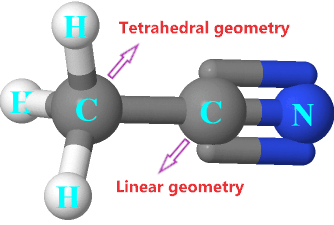## CH3CN molecular geometry

With respect to the left side carbon (C1)

The left side of carbon is surrounding by three hydrogen atoms and one right carbon atom i.e. means it has 4 regions of electron density and no lone pair present on it.

So, it implies tetrahedral geometry. You can also check its molecular geometry through the AXN method.

AXN notation for left side carbon:

• A denotes the central atom, therefore, A = Carbon
• X denotes the bonded atoms to the central atom, three hydrogens, and one right side carbon is bonded to it, therefore, X = 4
• N denotes the lone pair on the central atom, according to the CH3CN lewis structure, No lone pair is present on the left side carbon. Hence, N = 0

So, the AXN generic formula for the carbon (C1) becomes AX4.

As per the VSEPR chart, if a central atom is attached with four bonded atoms and has no lone pair then the molecular geometry with respect to that central atom is tetrahedral in nature, and electron geometry is also tetrahedral.

With respect to the right side carbon (C2)

The right side of carbon is surrounding by only two atoms (one is nitrogen and another one is left side carbon) i.e. means it has 2 regions of electron density and no lone pair present on it.

So, it implies linear geometry. You can also check its molecular geometry through the AXN method.

AXN notation for right side carbon:

• A denotes the central atom, therefore, A = Carbon
• X denotes the bonded atoms to the central atom, one nitrogen, and one left side carbon is bonded to it, therefore, X = 2
• N denotes the lone pair on the central atom, according to the CH3CN lewis structure, No lone pair is present on the right side carbon. Hence, N = 0

So, the AXN generic formula for the carbon (C2) becomes AX2.

As per the VSEPR chart, if a central atom is attached with two bonded atoms and has no lone pair then the molecular geometry with respect to that central atom is linear in nature, and electron geometry is also linear.## Hybridization of CH3CN

Let’s find the hybridization of CH3CN through the steric number of its central atom(C1 and C2).

“Steric number is the addition of a total number of bonded atoms around a central atom and the lone pair present on it.”

∴ Steric number of left side carbon atom(C1)= (Number of bonded atoms attached to C1 + Lone pair on C1)

According to the CH3CN lewis structure, the left side carbon atom (C1) is bonded to three hydrogen atoms, one right side carbon(C2), and no lone pair present on it.

∴ Steric number of carbon atom(C1) = (4 + 0) = 4

So, the steric number of 4 implies the Sp3 hybridization on the left side carbon atom(C1).

∴ Steric number of right side carbon atom(C2)= (Number of bonded atoms attached to C2 + Lone pair on C2)

According to the CH3CN lewis structure, the right side carbon atom (C2) is bonded to one nitrogen atom, one left side carbon(C1), and no lone pair present on it.

∴ Steric number of carbon atom(C2) = (2 + 0) = 2

So, the steric number of 2 implies the Sp hybridization on the right side carbon atom(C2).

 Steric number Hybridization 1 S 2 Sp 3 Sp² 4 Sp³ 5 Sp³d 6 Sp³d²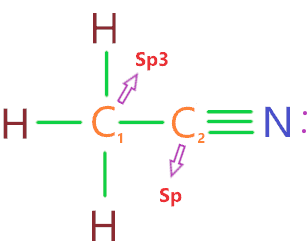## The bond angle of CH3CN

“The left carbon will have 109.5 bond angles and the right carbon will have 180 bond angles.”Image credit

## Is CH3CN polar or nonpolar?

CH3CN is a polar aprotic solvent means it doesn’t have an acidic proton but has the ability to dissolve ions in water.

Polar aprotic solvents contain no hydrogen atoms connected directly to an electronegative atom and they are not capable of hydrogen bonding.

Acetonitrile is not capable of hydrogen bonding in water, still, it is highly polar in nature with a dipole moment higher than 3.5 Debye. CH3CN has a very strong dipole-dipole force and is highly polar than water and alcohols.

Why CH3CN is polar? The nitrile functional group(C≡N) is polarized, because of the greater difference of electronegativity between carbon(2.55) and nitrogen(3.04), and the fact that a triple bond binds these 2 atoms. The consequence of this is the quite strong dipole moment (almost more than 3.5 Debye) in the CH3CN molecule.

As higher the dipole moment, the stronger is the polarity of that molecule.

## FAQ

 Why is the molecular geometry of CH3CN different with respect to the central atom? Because molecule geometry is dependent on the region of electron density i.e. bonded atoms and lone pairs electrons. As we see in CH3CN lewis’s structure, the left side carbon has 4 regions of electron density and the right side carbon is 2 regions of electron density.Hence, for 4 regions of electron density with no lone pair implies the tetrahedral geometry whereas 2 regions of electron density with zero lone pair implies the linear geometry.
 How many lone pair and bond pair electrons are present in the CH3CN lewis structure? There are only 2 lone pair electrons on the nitrogen atom and 14 bonded pair electrons(4 single bond and 1 triple bond) are present in the CH3CN lewis structure.

## Summary

• The total valence electron is available for drawing the CH3CN lewis structure is 16.
• There are two types of hybridization found in CH3CN molecules – (Sp3 and Sp).
• CH3CN is a polar aprotic solvent with a dipole moment of around 3.8 D and has a very strong dipole-dipole force.
• In the CH3CN molecule, only lone pairs and 7 bonded pairs are present.
• The molecular geometry of CH3CN with respect to C1 is tetrahedral and with respect to C2 is linear.
##### Subscribe to Blog via Email

Join 2 other subscribers

Share it...